Chapter 13 Ordinary Differential Equations

When studying objective phenomena, we often encounter such a type of mathematical problem, that is, the functional dependence relationship between a variable and other variables is unknown, but the unknown functional relationship and some of its derivatives (or differentials) are unknown. ) together with the independent variables are linked by a known equation , such an equation is called a differential equation . If the unknown function is univariate, the corresponding differential equation is called an ordinary differential equation ; if the unknown function is multivariate, then the corresponding The differential equations of are called partial differential equations .

This chapter introduces ordinary differential equations, and Chapter 14 introduces partial differential equations .

The main content of this chapter is to introduce several types of equations that can be solved analytically, such as some first-order differential equations, linear differential equations with constant coefficients, some higher-order differential equations and differential equations . For those equations that cannot be solved analytically, Introduce methods to study some properties of solutions (the general idea of ​​stability theory), or use some special methods to find approximate solutions of ordinary differential equations (mainly numerical solutions) .

§ 1   General Concepts of Differential Equations

A differential equation is a relation between independent variables , an unknown function and some of its derivatives :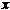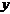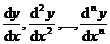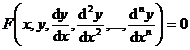[ Order of a Differential Equation ] The order of the highest derivative appearing in the equation is called the order of this differential equation .

For example :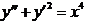is a second order ordinary differential equation .

[ degree of differential equation ] If the differential equation can be transformed into a rational integer for all derivatives, the degree of the highest derivative is called the degree of the differential equation . Not all differential equations have degrees . For example: a second-order second The second-order equation can be obtained after rationalization , but it is a second-order first-order equation, and the equation has no degree to say .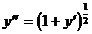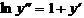[ Solution of Differential Equation ] The relationship between the variables that make an ordinary differential equation an identity is the solution of the ordinary differential equation . If the relationship is implicit, this solution is also called an integral . The method of finding the solution of a differential equation is also It can be called the integral method of the differential equation. The graph of each solution of the differential equation is also called the integral curve of the differential equation .

[ General solution of a differential equation ] If the number of independent arbitrary constants contained in the solution of a differential equation ( if a constant in a solution can take any value, it is called an arbitrary constant) is equal to the order of the differential equation, Then this solution is called the general solution of the differential equation . The general solution expression of a first-order differential equation contains an arbitrary constant independent of each other .[ Special solution of a differential equation ] Compared with the general solution, each solution of a differential equation is called a specific solution .

[ Initial value problem ] If an appropriate number of additional conditions are given at a certain value of the independent variable to determine the specific solution of the differential equation, then such a problem is called an initial value problem .

[ Boundary value problem ] If an appropriate number of additional conditions are given for more than one value of the independent variable to determine the particular solution of the differential equation, then such a problem is called a boundary value problem .